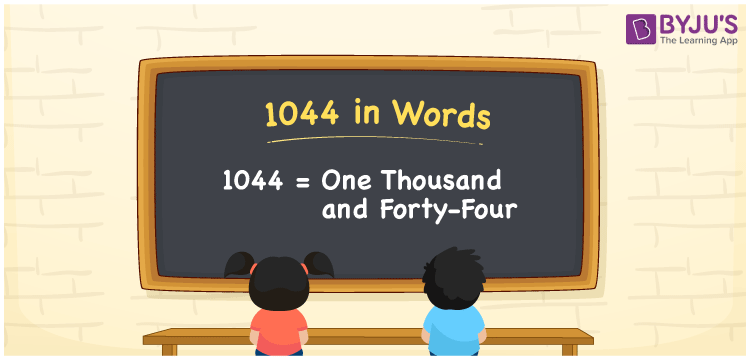# 1044 in words

1044 in words is written as One Thousand and Forty Four. 1044 represents the count or value. The article on Counting Numbers can give you an idea about count or counting. The number 1044 is used in expressions that relate to money, distance, length, year and others. Let us consider an example for 1044. ”The year One Thousand Four Hundred and Forty Four, was a leap year.”

 1044 in words One Thousand and Forty Four One Thousand and Forty Four in Numbers 1044

## 1044 in English Words## How to Write 1044 in Words?

We can convert 1044 to words using a place value chart. The number 1044 has 4 digits, so let’s make a chart that shows the place value up to 4 digits.

 Thousands Hundreds Tens Ones 1 0 4 4

Thus, we can write the expanded form as:

1 × Thousand + 0 × Hundred + 4 × Ten + 4 × One

= 1 × 1000 + 0 × 100 + 4 × 10 + 4 × 1

= 1044

= One Thousand and Forty Four.

1044 is the natural number that is succeeded by 1043 and preceded by 1045.

1044 in words – One Thousand and Forty Four.

Is 1044 an odd number? – No.

Is 1044 an even number? – Yes.

Is 1044 a perfect square number? – No.

Is 1044 a perfect cube number? – No.

Is 1044 a prime number? – No.

Is 1044 a composite number? – Yes.

## Solved Example

1. Write the number 1044 in expanded form

Solution: 1 × 1000 + 0 × 100 + 4 × 10 + 4 × 1

We can write 1044 = 1000 +000 +40 + 4

= 1 × 1000 + 0 × 100 + 4 × 10 + 4 × 1

## Frequently Asked Questions on 1044 in words

Q1

### How to write 1044 in words?

1044 in words is written as One Thousand and Forty Four.
Q2

### Is 1044 a perfect square number?

No. 1044 is not a perfect square number.
Q3

### Is 1044 a prime number?

No. 1044 is not a prime number.